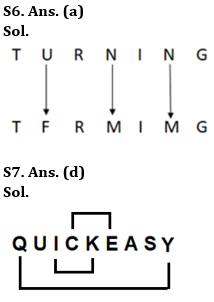Latest Banking jobs   »

# Reasoning Quizzes Quiz For IRDA AM 2023-13th May

Directions(1-5): Study the following series carefully & answer the questions given below.

E 7 & S 4 6 T # @ C 9 M N 1 ! D F 5 L Q \$ X 8

Q1. If all the symbols are dropped, then which element will be second to the right of eighth element from left end?
(a) 9
(b) M
(c) N
(d) 1
(e) D

Q2. How many symbols lies between 2nd number from left end and 2nd number from right end?
(a) 5
(b) 4
(c) 3
(d) 2
(e) 1

Q3. If all the numbers are dropped, then which element will be second to the left of twelfth element from left end?
(a) !
(b) F
(c) D
(d) N
(e) None of the above

Q4. How many such consonants are in the above arrangement, each of which is immediately preceded by a vowel or immediately followed by a symbol but not both?
(a) None
(b) One
(c) Two
(d) Three
(e) Four

Q5. How many such consonants are in the above arrangement, each of which is immediately followed by a vowel but not immediately preceded by a number?
(a) None
(b) Two
(c) Three
(d) Four
(e) Five

Q6. In a certain code language, if ‘PUNCTURE’ is coded as ‘PFNXTFRV’ and ‘FLAMES’ is coded as ‘FOANEH’, then what is the code for ‘TURNING’?
(a) TFRMIMG
(b) TFRMIGM
(c) TFRIMMG
(d) TFMRIMG
(e) None of these

Q7. How many such pairs of letters are there in the word ‘QUICKEASY’, each of which has as many letters between them as in the English alphabet (Both forward and backward)?
(a) None
(b) One
(c) Two
(d) Three
(e) More than three

Q8. If in the number ‘856347654’, 1 is subtracted from each even digit and 1 is added to each odd digit, then which digit is 7th from the left end?
(a) 5
(b) 8
(c) 4
(d) 3
(e) None of these

Q9. If in a certain way, ‘ABANDON’ is coded as ‘BCBOEPO’, then how ‘BABBLER’ will be coded in the same manner as?
(a) CBCCMFS
(b) CCBCFMS
(c) CFMSCCB
(d) FMSCBCC
(e) None of these

Q10. If it is possible to make a meaningful word, using the 2nd, 3rd, 6th and 7th letters of the word “TURMERIC’, then what is the second letter of the meaningful word (using each letter only once)? If no such meaningful word be formed, then mark the answer as ‘Z’. If more than one meaningful word can be formed then mark the answer as ‘K’.
(a) R
(b) I
(c) U
(d) K
(e) Z

Solutions

Solution (1-5):
S1. Ans. (c)
Sol. After dropping symbols: E 7 S 4 6 T C 9 M N 1 D F 5 L Q X 8
So, N will be second to the right of eighth element from left end. Hence, option (c) is correct answer.

S2. Ans. (c)
Sol. There are three symbols between 2nd number from left end and 2nd number from right end.

S3. Ans. (a)
Sol. After dropping numbers: E & S T # @ C M N ! D F L Q \$ X
So, ! will be second to the left of twelfth element from left end. Hence, option (a) is correct answer.

S4. Ans. (c)
Sol. Given Series – E 7 & S 4 6 T # @ C 9 M N 1 ! D F 5 L Q \$ X 8
So, there are two such consonants – T and Q.

S5. Ans. (a)
Sol. Given Series – E 7 & S 4 6 T # @ C 9 M N 1 ! D F 5 L Q \$ X 8
So, there is no such consonant.S8. Ans. (a)
Sol. If 1 is subtracted from each even digit and 1 is added to each odd digit – 765438563
Clearly, 5 is 7th digit from the left end.

S9. Ans. (a)
Sol. All the letters of the word are replaced by their succeeding letter according to English alphabetical series. Clearly, BABBLER will be coded as CBCCMFS.

S10. Ans. (e)
Sol. 2nd, 3rd, 6th and 7th letters of the word – U, R, R, I
Hence, no meaningful word can be formed.## FAQs

### How many sections are there in the IRDAI exam?

There are 4 sections in the IRDAI assistant manager online preliminary exam. They are Reasoning, English Language, General awareness, Quantitative aptitude.

#### Congratulations!Union Budget 2023-24: Free PDF